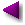Home | Java | OpticalRayTracer | * OpticalRayTracer Home Page OpticalRayTracer : Feedback OpticalRayTracer Technical (older, archived) OpticalRayTracer Technical Description Snell's Law CalculatorShare This Page
Snell's Law Calculator

A convenient JavaScript calculator for Snell's Law results

Current Version: 1.2 (11.19.2014)

Introduction | Calculator | Notes

(double-click any word to see its definition)

Introduction

Snell's Law describes the behavior of light when it encounters media having varying refractive indices. A refractive index is an expression of the ratio of the speed of light in a given medium versus its speed in a vacuum (with a refractive index of 1). I should add that light's speed doesn't actually change — it's more accurate to say that light takes a longer trip, with more delays, at full speed in glass than in air. Here's an example:Figure 1: Light wave moving from air to glass

Figure 1 should give readers an intuitive sense of why a change in the speed of light waves should also change their direction of travel. This is the basic idea behind lenses — it explains how they work. A curved lens surface can make a parallel light wave converge to a point, or diverge into an expanding cone, depending on whether the curve is convex or concave.

Here's the canonical expression of Snell's Law:

\begin{equation} \frac{\sin \theta_1}{\sin \theta_2} = \frac{v_1}{v_2} = \frac{n_2}{n_1} \end{equation}

Where:

• $\theta_1, \theta_2$ = angles (directions) taken by light waves.
• $v_1, v_2$ = light velocities in the respective media.
• $n_1, n_2$ = Unitless indices of refraction in the respective media.
The indices of refraction $n_1,n_2$ are related to the velocities $v_1,v_2$ and can be acquired from them. For a given velocity $v$: \begin{equation} n = \frac{c}{v} \end{equation}

Where $c$ = speed of light (299792458 m/s). Therefore as $v$ declines, $n$ becomes larger ($n$ is proportional to time delay). Here's an example — a typical measurement for $v$ in glass is 199861639 m/s. The index of refraction $n$ for this particular kind of glass is therefore:

\begin{equation} n = \frac{c}{v} = \frac{299792458}{199861639} = 1.5 \end{equation}

Equation (3) tells us that light requires 50% more time to pass through this specific glass.

Using Equation (1) as our basis and setting aside $v_1,v_2$, which are of little interest in optical work, here are the four equation forms by which useful results may be acquired:

\begin{equation} \theta_{1} = \operatorname{asin}{\left (\frac{n_{2}}{n_{1}} \sin{\left (\theta_{2} \right )} \right )} \end{equation} \begin{equation} \theta_{2} = \operatorname{asin}{\left (\frac{n_{1}}{n_{2}} \sin{\left (\theta_{1} \right )} \right )} \end{equation} \begin{equation} n_{1} = \frac{n_{2} \sin{\left (\theta_{2} \right )}}{\sin{\left (\theta_{1} \right )}} \end{equation} \begin{equation} n_{2} = \frac{n_{1} \sin{\left (\theta_{1} \right )}}{\sin{\left (\theta_{2} \right )}} \end{equation}

This means that, having three values from Equation (1), the fourth can be computed. This is the premise of the calculator below.

Calculator

Here's a JavaScript-generated graphic of the calculator's current result:

It seems this browser has no canvas support.

Change any of the values in the Entry column (you can use your mouse wheel), then press one of the buttons to acquire a result for the desired value:

Variable name Entry Compute Result
$n_1$
$n_2$
$\theta_1$
$\theta_2$

Details:

• To quickly experiment with different values, spin your mouse wheel over the entry fields.
• To become familiar with the calculator, enter values for $n_1,n_2$ and/or $\theta_1$, then press or the Enter key to compute $\theta_2$.
• To compute a result other than $\theta_2$, avoid pressing the Enter key on data entry — instead, enter values, then press the button associated with the desired value.
• To compute the critical angle for a given $n_1,n_2$ value pair:
• Enter $n_1,n_2$ values such that $n_1 \lt n_2$.
• Enter 90° for $\theta_1$ and press Enter (which will compute $\theta_2$).
• By definition, the computed $\theta_2$ value is the critical angle for the entered $n_1,n_2$ values.
• Angles should be expressed in degrees.
• Every effort has been made to simplify ongoing calculations. Results are copied to the entry column as well as the result column, so different hypotheses can be explored without laborious copying of results.
• Results such as "Infinity" and "NaN" can mean:
• A numeric-entry syntax error has been made — check your entries.
• if $n_1 \gt n_2$, meaning a ray of light is exiting a refractive medium, the conditions for total internal reflection may have been met.
• For sufficiently complex optical problems, the reader is encouraged to explore them with OpticalRayTracer, a free virtual optical bench.
Notes

Applied mathematics is becoming much easier in modern times. To prepare the code that solves the equations, and to include the equations in this article in LaTeX form, I needed to produce four practical forms of the Snell's Law equation. I could have sat down with pencil and paper and derived the four forms, but I'm too lazy for that. Instead I wrote and ran these few lines of Python:

from sympy import *

var('theta_1 theta_2 n_1 n_2')

snell = sin(theta_1) * n_1 - sin(theta_2) * n_2

for v in [theta_1,theta_2,n_1,n_2]:
pprint(solve(snell,v)[-1])


The result:

    ⎛n₂⋅sin(θ₂)⎞
asin⎜──────────⎟
⎝    n₁    ⎠

⎛n₁⋅sin(θ₁)⎞
asin⎜──────────⎟
⎝    n₂    ⎠

n₂⋅sin(θ₂)
──────────
sin(θ₁)

n₁⋅sin(θ₁)
──────────
sin(θ₂)


(If the above display box shows misaligned equation elements or missing Unicode characters, you must be using Microsoft's browser. The remedy is to download any other browser.)

A one-line change in the above code produces the LaTeX required for this article's equation renderings:

pprint(solve(snell,v)[-1]) -> print('%s = %s' % (v,latex(solve(snell,v)[-1])))


 Home | Java | OpticalRayTracer | * OpticalRayTracer Home Page OpticalRayTracer : Feedback OpticalRayTracer Technical (older, archived) OpticalRayTracer Technical Description Snell's Law CalculatorShare This Page